How to activate subcooling circuit in UNILAB COILS

To activate subcooling circuit in coil, you have to expande the "Subcooling Circ." on the left side of calculation mask:And then, flag the item "Subcooling circuit", so set the number of tubes for row, number of rows and related number of circuits: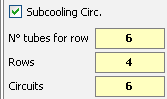How UNILAB COILS considers the subcooling circuitWhen you calculate a condensing coil with subcooling circuit,

the output capacity in the main calculation mask is related to the only condensing coil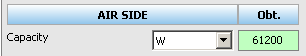the output capacity of the subcooling circuit is in the left panel of the subcooling circuit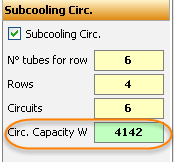instead the subcooling is the real subcooling degrees in the subcooling circuit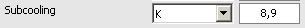the pressure drop is the sum of condensing coil and subcooling circuitIn the printout you will find 2 blocks of pages:The first block is related ONLY to the condensing coil, so you will find the output capacity, pressure drops and all others data. The subcooling will be 0K because the subcooling is calculated in the second block dedicated to the subcooling circuit:

FIRST BLOCK (CONDENSING COIL)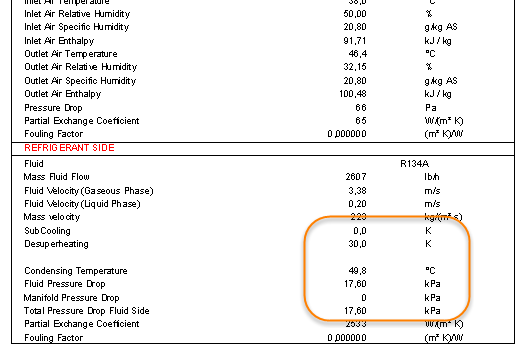SECOND BLOCK (SUBCOOLING CIRCUIT)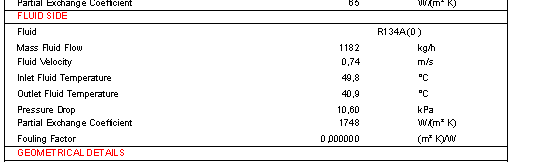So,

the total pressure drops will be: 17.60kPa (condensing coil) + 10.60kPa (subcooling circuit), total = 28.2kPa

the subcooling degress will be temperature difference between inlet and outlet of the liquid in the subcooling circuit, so 49.8°C - 40.9°C, total subcooling = 8.9K